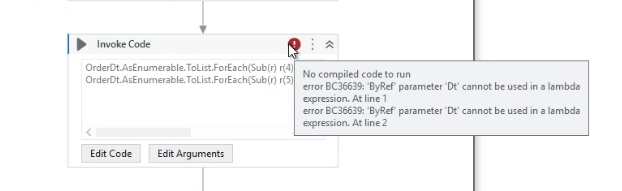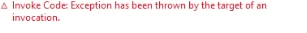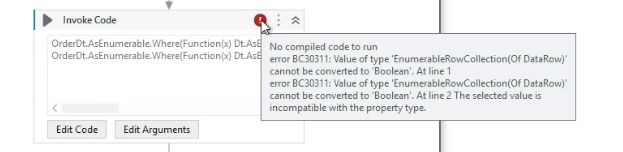# LinQ for 2 For Loops and 1 If loop

Hi Team,

I am having issue in writing a LINQ for a condition given below,

for each row in order_dt
{
For each row in dt
{
if order_dt(1)=dt(0)
{
order_dt(4)=dt(1)
order_dt(5)=dt(2)
}
}
}

@yash.choursia

For Each Row (orderRow) in order_dt
For Each Row (dtRow) in dt
If orderRow(“Column1”).ToString = dtRow(“Column0”).ToString
Assign orderRow(“Column4”) = dtRow(“Column1”)
Assign orderRow(“Column5”) = dtRow(“Column2”)
End If
End For Each Row
End For Each Row

Try this hope it helps

``````For Each Row in order_dt
For Each Row in dt
If order_dt(1) = dt(0)
Assign order_dt(4) = dt(1)
Assign order_dt(5) = dt(2)
``````

Hope it helps!!

@yash.choursia

Hi
what was the scenario you want to get for both the datatables

@yash.choursia

Please try this in invoke code…orderdt as in/out and dt as in

``````orderdt.AsEnumerable.ToList.ForEach(Sub(r) r(4) = dt.AsEnumerable.Where(Function(x) x(0).ToString=r(1).ToString).First()(1))
orderdt.AsEnumerable.ToList.ForEach(Sub(r) r(5) = dt.AsEnumerable.Where(Function(x) x(0).ToString=r(1).ToString).First()(2))
``````

cheers

1 Like

@yash.choursia

from orderRow in order_dt.AsEnumerable()
from dtRow in dt.AsEnumerable()
where orderRow.Field(1) == dtRow.Field(0)
select new
{
OrderDtRow = orderRow,
DtRow = dtRow
};

Cheers…!@yash.choursia

I think you gave dt as in/out…please give as only in parameter

That should fix the error

cheers

it is not giving compilation error. but while running it is giving error as:@yash.choursia

1. Open the locals panel…and then check the exception details from there please
2. does orderdt contain all dt values if not…please try this below one
``````orderdt.AsEnumerable.Where(function(x) dt.AsEnumerable.Where(function(y) y(0).ToString=x(1).ToString)).ToList.ForEach(Sub(r) r(4) = dt.AsEnumerable.Where(Function(x) x(0).ToString=r(1).ToString).First()(1))
orderdt.AsEnumerable.Where(function(x) dt.AsEnumerable.Where(function(y) y(0).ToString=x(1).ToString).ToList.ForEach(Sub(r) r(5) = dt.AsEnumerable.Where(Function(x) x(0).ToString=r(1).ToString).First()(2))
``````

cheers@yash.choursia

``````orderdt.AsEnumerable.Where(Function(x) dt.AsEnumerable.Any(Function(y) y(0).ToString=x(1).ToString)).ToList.ForEach(Sub(r) r(4) = dt.AsEnumerable.Where(Function(x) x(0).ToString=r(1).ToString).First()(1))
orderdt.AsEnumerable.Where(Function(x) dt.AsEnumerable.Any(Function(y) y(0).ToString=x(1).ToString)).ToList.ForEach(Sub(r) r(5) = dt.AsEnumerable.Where(Function(x) x(0).ToString=r(1).ToString).First()(2))
``````

cheers

1 Like

Could also check by having a mixture of Linq and `For Each`, as we have to perform an Update, we would require to use the `For Each Row` for `order_dt` and the Inner For Each could be eliminated with a Linq Expression fetching the matching values.

Here, `dr_Array` is a variable of the type Array of DataRow.

This topic was automatically closed 3 days after the last reply. New replies are no longer allowed.